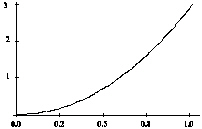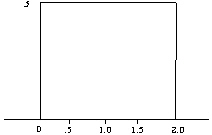Name:    AP Statistics - Chapter 2 MC Study Guide

Multiple Choice
Identify the choice that best completes the statement or answers the question.

1.

The heights of American men aged 18 to 24 are approximately normally distributed with mean 68 inches and standard deviation 2.5 inches.  Only about 5% of young men have heights outside the range
 a. 65.5 inches to 70.5 inches b. 63 inches to 73 inches c. 60.5 inches to 75.5 inches d. 58 inches to 78 inches e. none of the above

2.

For the density curve shown below, which statement is true?a. The density curve is symmetric. b. The density curve is skewed right. c. The area under the curve between 0 and 1 is 1. d. The density curve is normal. e. None of the above is correct.

3.

For the density curve shown below, which statement is true?a. The mean and median are equal. b. The mean is greater than the median. c. The mean is less than the median. d. The mean could be either greater than or less than the median. e. None is the above is correct.

4.

The area under the standard normal curve corresponding to –0.3<Z<1.6 is
 a. 0.3273 b. 0.4713 c. 0.5631 d. 0.9542 e. None of the above

5.

Suppose that sixteen-ounce bags of chocolate chips cookies are produced with an actual mean weight of 16.1 ounces and a standard deviation of 0.1 ounce. The percentage of bags that will contain between 16.0 and 16.1 ounces is
 a. 10 b. 16 c. 34 d. 68 e. none of the above

6.

A company produces packets of soap powder labeled "Giant Size 32 Ounces." The actual weight of soap powder in a box has a normal distribution with a mean of 33 oz. and a standard deviation of 0.8 oz. What proportion of packets are underweight (i.e., weigh less than 32 oz.)?
 a. 0.159 b. 0.212 c. 0.106 d. 0.841 e. 0.115

7.

For the density curve below, what percent of the observations lie between 0.5 and 1.2?a. 25% b. 35% c. 50% d. 68% e. 70%

8.

If the heights of 99.7% of American men are between 5'0" and 7'0", what is your estimate of the standard deviation of the height of American men?
 a. 1” b. 3” c. 4” d. 6” e. 12”

9.

Suppose that the distribution of math SAT scores from your state this year is normally distributed with mean 480 and standard deviation 100 for males, and mean 440 and standard deviation 120 for females. If someone who scores 780 or higher on math SAT can be considered a genius, what is the proportion of geniuses among the male SAT takers?
 a. 26% b. 13% c. 3% d. 1.3% e. 0.13%

10.

The average yearly snowfall in Chillyville is normally distributed with a mean of 55 inches.  If the snowfall in Chillyville exceeds 60 inches in 15% of the years, what is the standard deviation?
 a. 4.81 inches b. 5.18 inches c. 6.04 inches d. 8.93 inches e. The standard deviation cannot be computed from the given information.